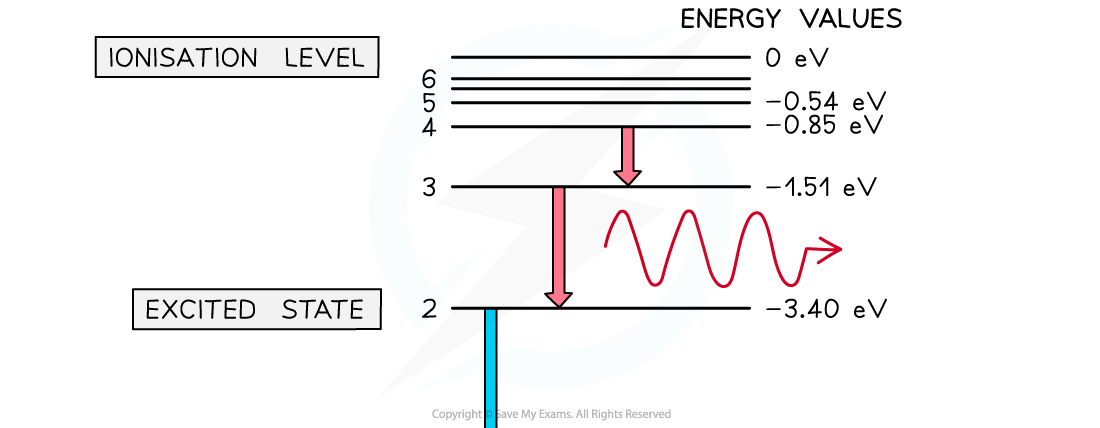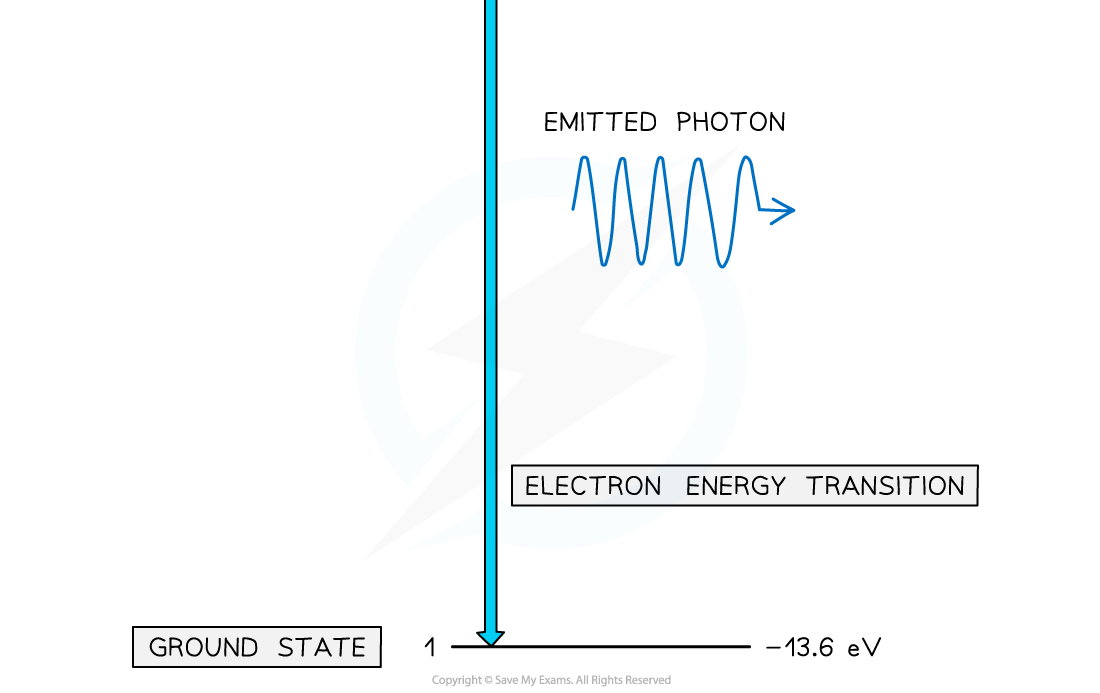# IB DP Physics: HL复习笔记7.1.1 Discrete Energy Levels

### Discrete Energy Levels

#### The Photon Model

• Photons are fundamental particles that make up all forms of electromagnetic radiation
• A photon is a massless “packet” or a “quantum” of electromagnetic energy
• What this means is that the energy is not transferred continuously but as discrete packets of energy
• In other words, each photon carries a specific amount of energy, and transfers this energy all in one go

#### Calculating Photon Energy

• The energy of a photon can be calculated using the formula:

E = hf

• Using the wave equation, energy can also be equal to:• Where:
• E = energy of the photon (J)
• h = Planck's constant (J s)
• c = the speed of light (m s-1)
• f = frequency (Hz)
• λ = wavelength (m)
• This equation tells us:
• The higher the frequency of EM radiation, the higher the energy of the photon
• The energy of a photon is inversely proportional to the wavelength
• A long-wavelength photon of light has a lower energy than a shorter-wavelength photon

#### Atomic Energy Levels

• Electrons in an atom can have only certain specific energies
• These energies are called electron energy levels
• They can be represented as a series of stacked horizontal lines increasing in energy
• Normally, electrons occupy the lowest energy level available, this is known as the ground state
• Electrons can gain energy and move up the energy levels if it absorbs energy either by:
• Collisions with other atoms or electrons
• Absorbing a photon
• A physical source, such as heat
• This is known as excitation, and when electrons move up an energy level, they are said to be in an excited state
• If the electron gains enough energy to be removed from the atom entirely, this is known as ionisation
• When an electron returns to a lower energy state from a higher excited state, it releases energy in the form of a photonElectron energy levels in atomic hydrogen. Photons are emitted when an electron moves from a higher energy state to a lower energy state